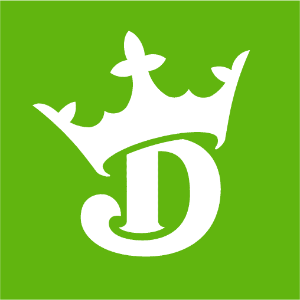DraftKings 2020 Defensive Player of the Year Odds
+600
6 to 1T.J. Watt
8.5% implied probability

+800
8 to 1Aaron Donald
6.6% implied probability

+1000
10 to 1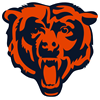Khalil Mack
5.4% implied probability

+1400
14 to 1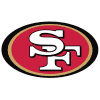Nick Bosa
4.0% implied probability

No changes have been recorded yet.
+1400
14 to 1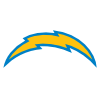Joey Bosa
4.0% implied probability

+1400
14 to 1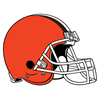Myles Garrett
4.0% implied probability

+1600
16 to 1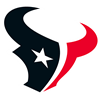J.J. Watt
3.5% implied probability

+2000
20 to 1Stephon Gilmore
2.8% implied probability

+2100
21 to 1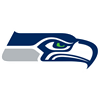Jamal Adams
2.7% implied probability

+2200
22 to 1Chase Young
2.6% implied probability

+2500
25 to 1Chandler Jones
2.3% implied probability

+2500
25 to 1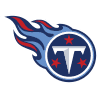Jadeveon Clowney
2.3% implied probability

+2500
25 to 1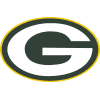Za'Darius Smith
2.3% implied probability

+2500
25 to 1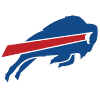Tre'Davious White
2.3% implied probability

+3000
30 to 1Bobby Wagner
1.9% implied probability

+3300
33 to 1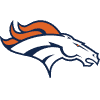Von Miller
1.7% implied probability

+3300
33 to 1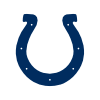Darius Leonard
1.7% implied probability

No changes have been recorded yet.
+3300
33 to 1Jalen Ramsey
1.7% implied probability

+3300
33 to 1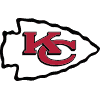Tyrann Mathieu
1.7% implied probability

+5000
50 to 1Chris Jones
1.2% implied probability

No changes have been recorded yet.
+5000
50 to 1Minkah Fitzpatrick
1.2% implied probability

+5000
50 to 1Melvin Ingram
1.2% implied probability

+5000
50 to 1Cameron Jordan
1.2% implied probability

+5000
50 to 1Jaire Alexander
1.2% implied probability

+6000
60 to 1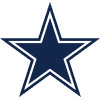DeMarcus Lawrence
1.0% implied probability

+6000
60 to 1Shaquil Barrett
1.0% implied probability

+6600
66 to 1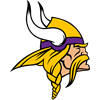Yannick Ngakoue
0.9% implied probability

+6600
66 to 1Danielle Hunter
0.9% implied probability

+6600
66 to 1Marshon Lattimore
0.9% implied probability

No changes have been recorded yet.
+6600
66 to 1DeForest Buckner
0.9% implied probability

+6600
66 to 1Bradley Chubb
0.9% implied probability

+6600
66 to 1Calais Campbell
0.9% implied probability

+7000
70 to 1Leighton Vander Esch
0.8% implied probability

No changes have been recorded yet.
+7000
70 to 1Marcus Peters
0.8% implied probability

No changes have been recorded yet.
+7000
70 to 1Preston Smith
0.8% implied probability

+8000
80 to 1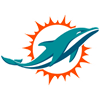Byron Jones
0.7% implied probability

+8000
80 to 1Earl Thomas
0.7% implied probability

No changes have been recorded yet.
+8000
80 to 1Richard Sherman
0.7% implied probability

+8000
80 to 1Devin White
0.7% implied probability

+8000
80 to 1Eric Kendricks
0.7% implied probability

+10000
100 to 1Kevin Byard
0.6% implied probability

+10000
100 to 1Darnell Savage
0.6% implied probability

No changes have been recorded yet.
+10000
100 to 1Marcus Davenport
0.6% implied probability

+10000
100 to 1Devin McCourty
0.6% implied probability

+10000
100 to 1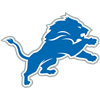Trey Flowers
0.6% implied probability

+10000
100 to 1Cameron Heyward
0.6% implied probability

+10000
100 to 1Vita Vea
0.6% implied probability

No changes have been recorded yet.
+10000
100 to 1Justin Houston
0.6% implied probability

No changes have been recorded yet.
+10000
100 to 1Adrian Amos
0.6% implied probability

No changes have been recorded yet.
+10000
100 to 1Arik Armstead
0.6% implied probability

No changes have been recorded yet.
+10000
100 to 1Akiem Hicks
0.6% implied probability

+10000
100 to 1Derwin James
0.6% implied probability

+10000
100 to 1Kevin King
0.6% implied probability

No changes have been recorded yet.
+10000
100 to 1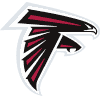Grady Jarrett
0.6% implied probability

No changes have been recorded yet.
+10000
100 to 1Shaq Lawson
0.6% implied probability

No changes have been recorded yet.
+10000
100 to 1Deion Jones
0.6% implied probability

+15000
150 to 1Isaiah Simmons
0.4% implied probability

No changes have been recorded yet.
+15000
150 to 1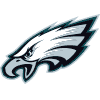Brandon Graham
0.4% implied probability

+15000
150 to 1Ed Oliver
0.4% implied probability

No changes have been recorded yet.
+15000
150 to 1Rashan Gary
0.4% implied probability

No changes have been recorded yet.
+15000
150 to 1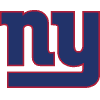Dexter Lawrence
0.4% implied probability

No changes have been recorded yet.
+15000
150 to 1Devin Bush
0.4% implied probability

No changes have been recorded yet.
+15000
150 to 1Everson Griffen
0.4% implied probability

+15000
150 to 1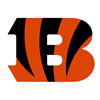Geno Atkins
0.4% implied probability

No changes have been recorded yet.
+15000
150 to 1Dante Fowler
0.4% implied probability

+15000
150 to 1Frank Clark
0.4% implied probability

No changes have been recorded yet.
+15000
150 to 1Landon Collins
0.4% implied probability

No changes have been recorded yet.
+15000
150 to 1Dee Ford
0.4% implied probability

No changes have been recorded yet.
+15000
150 to 1Fletcher Cox
0.4% implied probability

+15000
150 to 1Jaylon Smith
0.4% implied probability

+15000
150 to 1Harrison Smith
0.4% implied probability

No changes have been recorded yet.
+20000
200 to 1Quinton Dunbar
0.3% implied probability

No changes have been recorded yet.
+20000
200 to 1Jabrill Peppers
0.3% implied probability

No changes have been recorded yet.
+20000
200 to 1Darius Slay
0.3% implied probability

+20000
200 to 1Malcolm Jenkins
0.3% implied probability

No changes have been recorded yet.
+20000
200 to 1Christian Wilkins
0.3% implied probability

No changes have been recorded yet.
+20000
200 to 1Josh Allen
0.3% implied probability

No changes have been recorded yet.
+20000
200 to 1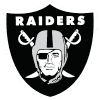Clelin Ferrell
0.3% implied probability

+20000
200 to 1Quinnen Williams
0.3% implied probability

+20000
200 to 1Maxx Crosby
0.3% implied probability

No changes have been recorded yet.
+20000
200 to 1Leonard Williams
0.3% implied probability

+20000
200 to 1Anthony Barr
0.3% implied probability

+20000
200 to 1Jamie Collins
0.3% implied probability